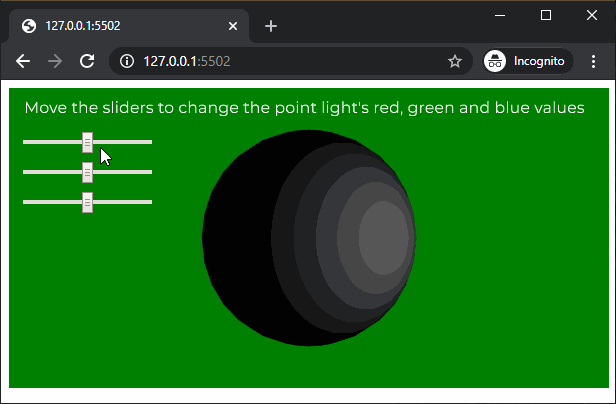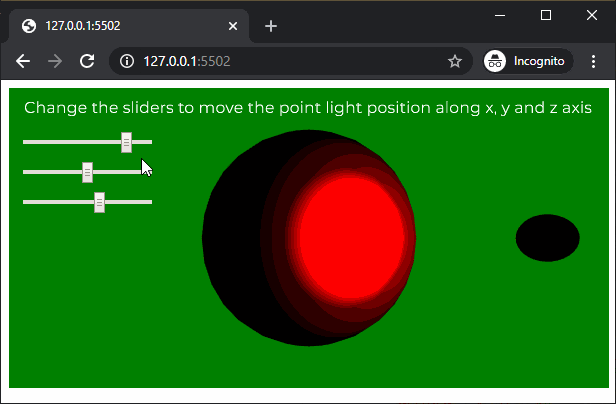# p5.js | pointLight() Function

The pointLight() function in p5.js is used to create a point lights with the specified color and position in the scene. A scene can have a maximum of 5 active point lights at a time.

Syntax:

`pointLight( v1, v2, v3, x, y, z )`

OR

`pointLight( v1, v2, v3, position )`

OR

`pointLight( color, x, y, z )`

OR

`pointLight( color, position )`

Parameters: This function accept eight parameters as mentioned above and described below:

• v1: It is a number which determines the red or hue value relative to the current color range.
• v2: It is a number which determines the green or saturation value relative to the current color range.
• v3: It is a number which determines the blue or brightness value relative to the current color range.
• x: It is a number which determines the x axis position of the light.
• y: It is a number which determines the y axis position of the light.
• z: It is a number which determines the z axis position of the light.
• position: It is p5.Vector which defines the position of the light.
• color: It is a color string or p5.Color which defines the color of the point light.

Below example illustrates the pointLight() function in p5.js:

Example 1:

 `let newFont; ` `let directionalLightEnable = ``false``; ` ` `  `function` `preload() { ` `  ``newFont = loadFont(``'fonts/Montserrat.otf'``); ` `} ` ` `  `function` `setup() { ` `  ``createCanvas(600, 300, WEBGL); ` `  ``textFont(newFont, 16); ` ` `  `  ``redSlider = createSlider(0, 255, 128, 1); ` `  ``redSlider.position(20, 50); ` ` `  `  ``greenSlider = createSlider(0, 255, 128, 1); ` `  ``greenSlider.position(20, 80); ` ` `  `  ``blueSlider = createSlider(0, 255, 128, 1); ` `  ``blueSlider.position(20, 110); ` `} ` ` `  `function` `draw() { ` `  ``background(``'green'``); ` `  ``text(``"Move the sliders to change the point light's red, "``+ ` `                        ``"green and blue values"``, -285, -125); ` `  ``noStroke(); ` ` `  `  ``redValue = redSlider.value(); ` `  ``greenValue = greenSlider.value(); ` `  ``blueValue = blueSlider.value(); ` ` `  `  ``// Create a point light with the selected light color ` `  ``pointLight(redValue, greenValue, blueValue, 100, 0, 150); ` ` `  `  ``ambientMaterial(255); ` ` `  `  ``// Create the sphere on which the point light will work ` `  ``sphere(100); ` `} `

Output:Example 2:

 `let newFont; ` `let directionalLightEnable = ``false``; ` ` `  `function` `preload() { ` `  ``newFont = loadFont(``'fonts/Montserrat.otf'``); ` `} ` ` `  `function` `setup() { ` `  ``createCanvas(600, 300, WEBGL); ` `  ``textFont(newFont, 16); ` ` `  `  ``xPosSlider = createSlider(-150, 150, 100, 1); ` `  ``xPosSlider.position(20, 50); ` ` `  `  ``yPosSlider = createSlider(-300, 300, 0, 1); ` `  ``yPosSlider.position(20, 80); ` ` `  `  ``zPosSlider = createSlider(0, 250, 150, 1); ` `  ``zPosSlider.position(20, 110); ` ` `  `} ` ` `  `function` `draw() { ` `  ``background(``'green'``); ` `  ``text(``"Change the sliders to move the point light position "``+ ` `                          ``"along x, y and z axis"``, -285, -125); ` `  ``noStroke(); ` ` `  `  ``xPositionValue = xPosSlider.value(); ` `  ``yPositionValue = yPosSlider.value(); ` `  ``zPositionValue = zPosSlider.value(); ` ` `  `  ``// Create a point light at the selected location ` `  ``pointLight(255, 0, 0, xPositionValue, yPositionValue, zPositionValue); ` ` `  `  ``// Create a sphere to show the demonstrate ` `  ``// of the point light location ` `  ``translate(xPositionValue, yPositionValue, zPositionValue); ` `  ``sphere(10); ` `  ``translate(-xPositionValue, -yPositionValue, -zPositionValue); ` ` `  `  ``specularMaterial(255); ` ` `  `  ``// Create the sphere on which the point light will work ` `  ``sphere(100); ` `} `

Output:Online editor: https://editor.p5js.org/My Personal Notes arrow_drop_upCheck out this Author's contributed articles.

If you like GeeksforGeeks and would like to contribute, you can also write an article using contribute.geeksforgeeks.org or mail your article to contribute@geeksforgeeks.org. See your article appearing on the GeeksforGeeks main page and help other Geeks.

Please Improve this article if you find anything incorrect by clicking on the "Improve Article" button below.

Article Tags :

Be the First to upvote.

Please write to us at contribute@geeksforgeeks.org to report any issue with the above content.